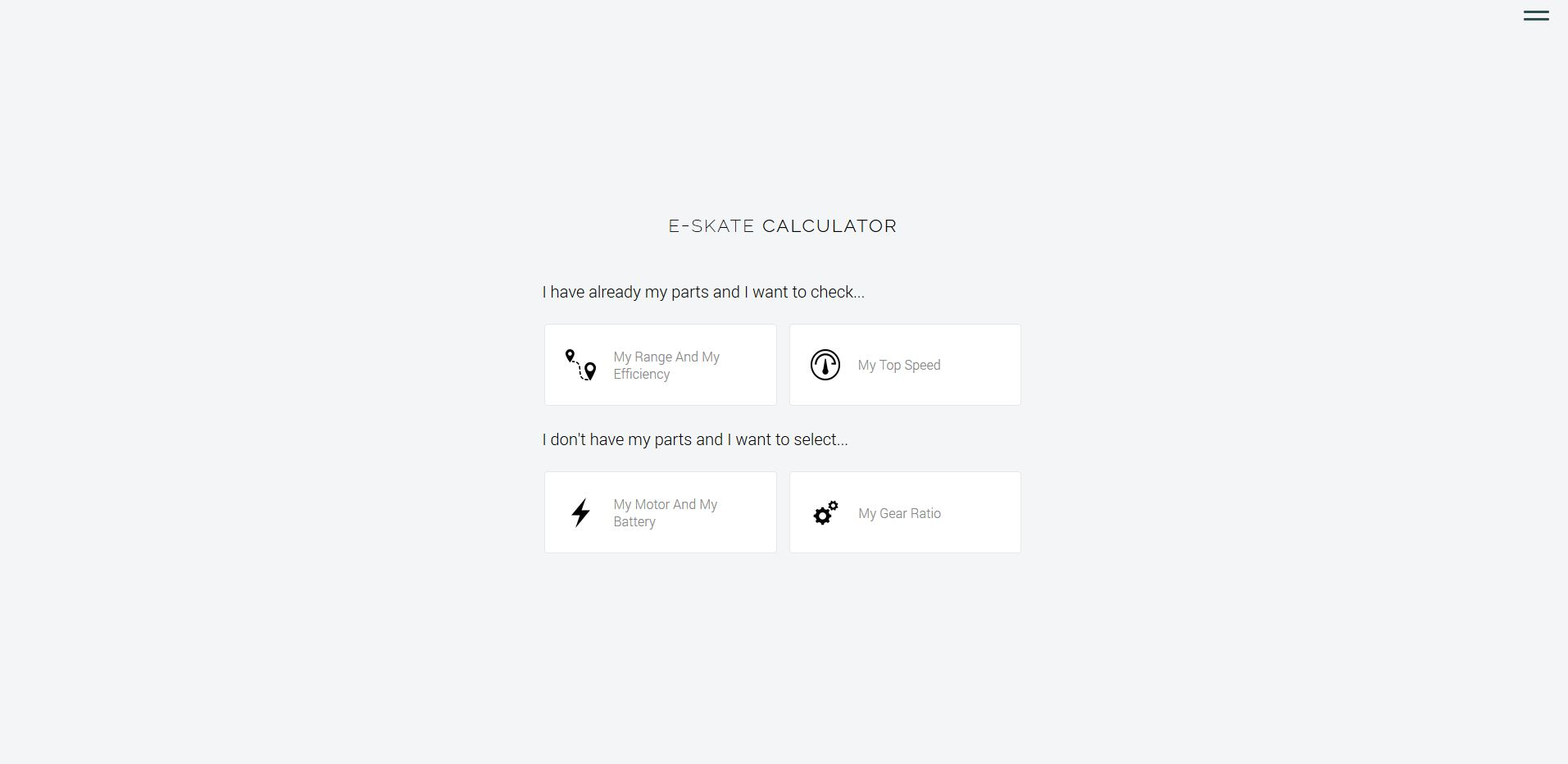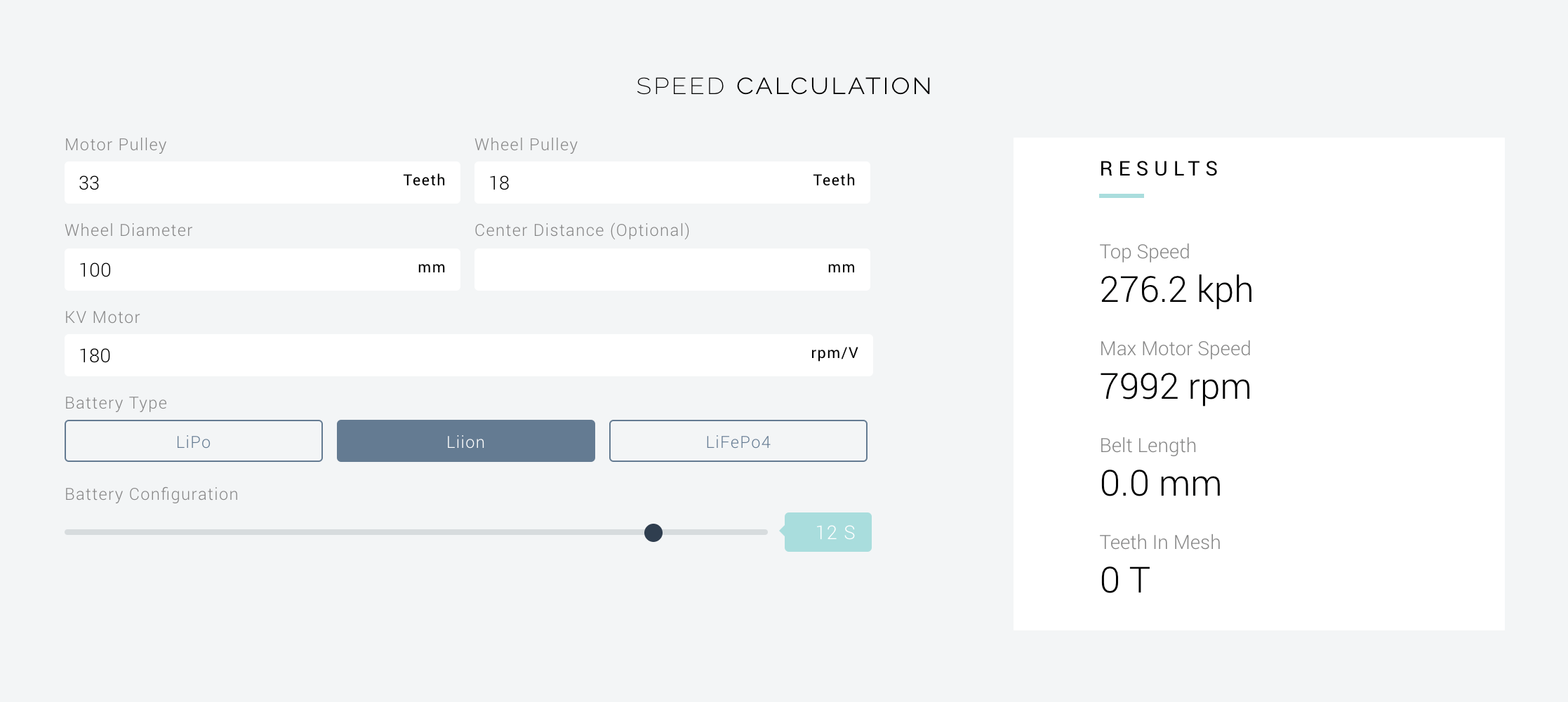# ND | Calculator

I have built a calculator based on a physical models for parts selection, or to check setup performances:

https://norde.cc/calculator.php

For the time being, you can determine your motor power, capacity, and gear ratio based on your requirements. You can also check your range, your system efficiency and your top speed based on your parts. Here is a screenshot:Fields explanation

## Power calculation

• Rider weight: Your weight in kg
• Speed: The average speed you would like to achieve
• Start time: Time to achieve your average speed
• Flat distance: Range you would like to achieve on flat road
• Climbing distance: Range you would like to achieve on slope
• Motor efficiency: The efficiency of your motor
• Motor configuration: Dual or single
• Battery type: Chemical technology of your battery
• Battery configuration: Number of cells of your battery

## Drivetrain calculation

• Motor pulley: Number of teeth for your motor pulley
• Wheel pulley: Number of teeth for your wheel pulley
• Wheel diameter: Diameter of the wheel (in mm)
• Center distance (Optional): Center distance between your motor pulley and your wheel pulley
• KV Motor: Motor kv constant
• Battery type: Battery technology
• Battery configuration: Number of battery cells

To perform a calculation, you can press “Enter” on a field.

Enjoy!

10 Likes

Thanks for your contribution, but I don’t really know how to use itI have added some explanation on how to complete the form. You can press enter to perform calculation. Does it make more sense now?

The range calculation makes my 12S6P dual at 25KM/h appear as a 100+KM range one if I only go flat.I really doubt it. Even adding inclines I still get 60KM of combined range. Never ever is it going to be so.1 Like

I am waiting for more feedbacks like this to find parameters that fit everyone the best. For the time being, you may want to put the motor efficiency to 50% which may give you a good error margin.

As it is a dual pneumatics setup I expect no more than 30-40km if I hold 25km/h and never do some very fast accelerations. What is your loss constant for dual motors?

I don’t use a loss constant. If you are interested in power calculation, here are the formulas that I used :

The process is first to calculate forces, then power, voltage, current and finally capacity.

During a ride, we will consider two steps:

• Acceleration phase
• Nominal phase (flat or slope)

## Force

Acceleration phase

Let’s consider the acceleration phase. We take into account three forces:

Rolling resistance: f_roll = f.m.g

• f: friction coefficient for urethane on asphalt, f=0.015
• m: rider mass in kg
• g: acceleration of gravity, g=9.81m/s²

Aerodynamic resistance: f_aero = 1/2.ρ.Sx.V²

• ρ: air density ρ = 1,204 kg/m^3
• Sx: drag surface, for an average size rider I estimated Sx = 0.6m²
• V: average speed in m/s

Inertia: F_ie = m.a

• m: rider mass in kg
• a: acceleration in m/s²

The acceleration is expressed:

Acceleration: a = V/t_start

• V: average speed in m/s
• t_start: start time in s

Total forces: F_acc = f_roll + f_aero + f_ie

Nominal phase on flat

There are only two forces left:

Rolling resistance: f_roll = f.m.g

Aerodynamic resistance: f_aero = 1/2.ρ.Sx.V²

Total forces: F_flat = f_roll + f_aero

Nominal phase on slope

Rolling resistance: f_roll = f.m.g.cos ∝

Aerodynamic resistance: f_aero = 1/2.ρ.Sx.V²

Weight: F_weight = m.g.sin∝

Total forces: F_slope = f_roll + f_aero + F_weight

## Power

To convert forces into mechanical power that applies on the wheel, we use: P_meca_wheel = F.V

• F: total forces in N
• V: average speed in m/s

To get the mechanical power that applies to the pulley motor, we use:

P_meca_motor = P_meca_wheel/µ

• µ: efficiency of the drivetrain, for a pulley-belt setup we consider: µ=80%

Finally, the electrical power that needs to be provided to the motor is expressed:

P_elec_motor = P_meca_motor/e

• e: efficiency of the motor

Then, we calculate P_elec_acc, P_elec_flat, P_elec_slope for each phase.

Dual/Single If the setup is dual, we divided P_elec by two.

Displayed power The displayed power is the max power among each power phases

## Voltage

• For LiPo/Li-ion technology: U=3.7V*S, with S the number of cells
• For LiFePo4 technology: U=3.2V*S

## Current

I = P_elec/U. We get I_acc, I_flat, I_slope for each phase.

The average current is expressed:

I_avg = p.I_slope + (1-p).I_flat

• p: percentage of route in slope.

p = d_slope/(d_slope+d_flat)=d_slope/d_total

• d_flat: Range on flat road (flat distance)
• d_slope: Range on slope (climbing distance)
• d_total: Total distance

## Capacity

C = I_avg.t

• t : travelling time

t = V/d_total

• V: average speed
• d: total distance
4 Likes

I have just noticed that you said you are on pneumatics. I have integrated this yet. Calculations are made for urethane on asphalt.

I need more data for pneumatics setup, but I am working on it

5 Likes

Perfect dude. I would love to see the most science-inducing calculator around. Keep up the good work!

2 Likes

You can use an efficiency modifier in your formula where thane is calculated at 90% and pnumatics are calculated at 75% (as an example).

Something like this…

Wh / 30 * efficiency % = range in Miles

Wh / 30 * 1.6 * efficiency % = range in KM

Thank you for your link. Yes, for the time being people could do something like that by moving the “motor efficiency” parameter, but I would like to find the coefficient of friction for pneumatics on asphalt. I will just change that and the total weight of (rider + board).

Just in case, because I think it is not obvious, but there is a second page with drivetrain calculation. You can navigate through the buttons on the left.

1 Like

The new version is online!

## Calculator Update

Now you can use a mode to check what kind of performances you can except with your parts, and another one to choose your parts.

If you have any suggestion, let me know!

1 Like

Is there an option to use imperial? Also is this supposed to happen or am I missing something?2 Likes

@sofu Reverse Wheel and motor pulley

3 Likes

I’m dumb3 Likes

I can add imperial, but it will take me more time than the other updates. Do you need it for all the fields, or only for specific ones, like top speed?

i guess only specific ones like speed related stuff

Torque would be coolEmtb folks like that stat, I would imagine

Sure, I plan to add torque as soon as possibleNew update: No torque or imperial, but I have added a belt length and a battery calculators:

https://norde.cc/calculator.php

Also, for the power calculator, I have divided the battery capacity by 80%, since only 80% of a lithium battery is usable.